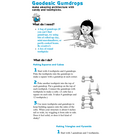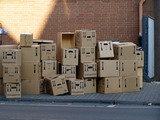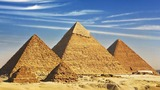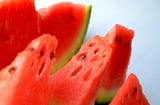Updating search results...

# 10 Results

View
Selected filters:
• cubesUnrestricted Use
CC BY
Rating
0.0 stars

Family facing 6th Grade math unit focusing on area and surface area.

Subject:
Geometry
Mathematics
Material Type:
Unit of Study
Provider:
Illustrative Mathematics
05/11/2020Unrestricted Use
CC BY
Rating
0.0 stars

In this unit, students learn to find areas of polygons by decomposing, rearranging, and composing shapes. They learn to understand and use the terms “base” and “height,” and find areas of parallelograms and triangles. Students approximate areas of non-polygonal regions by polygonal regions. They represent polyhedra with nets and find their surface areas.

Subject:
Geometry
Mathematics
Material Type:
Unit of Study
Provider:
Illustrative Mathematics
05/11/2020Unrestricted Use
CC BY
Rating
0.0 stars

Student facing 6th Grade math unit focusing on area and surface area.

Subject:
Geometry
Mathematics
Material Type:
Unit of Study
Provider:
Illustrative Mathematics
05/11/2020Conditional Remix & Share Permitted
CC BY-NC-SA
Rating
0.0 stars

This hands-on activity shows you how to build basic architectural shapes out of toothpicks and gumdrops. Learners explore how different shapes are more stable than others, and are introduced to ideas about "stretching and squashing"--that is, about tension and compression.

Subject:
Applied Science
Architecture and Design
Material Type:
Activity/Lab
Provider:
Exploratorium
Author:
Ellen Klages
Linda Shore
Pat Murphy
The Exploratorium
12/07/1998Conditional Remix & Share Permitted
CC BY-NC
Rating
0.0 stars
Rating
0.0 stars
Subject:
Mathematics
Material Type:
Full Course
Provider:
Pearson
10/06/2016Conditional Remix & Share Permitted
CC BY-NC
Rating
0.0 stars

Surface Area and Volume

Type of Unit: Conceptual

Prior Knowledge

Students should be able to:

Identify rectangles, parallelograms, trapezoids, and triangles and their bases and heights.
Identify cubes, rectangular prisms, and pyramids and their faces, edges, and vertices.
Understand that area of a 2-D figure is a measure of the figure's surface and that it is measured in square units.
Understand volume of a 3-D figure is a measure of the space the figure occupies and is measured in cubic units.

Lesson Flow

The unit begins with an exploratory lesson about the volumes of containers. Then in Lessons 2–5, students investigate areas of 2-D figures. To find the area of a parallelogram, students consider how it can be rearranged to form a rectangle. To find the area of a trapezoid, students think about how two copies of the trapezoid can be put together to form a parallelogram. To find the area of a triangle, students consider how two copies of the triangle can be put together to form a parallelogram. By sketching and analyzing several parallelograms, trapezoids, and triangles, students develop area formulas for these figures. Students then find areas of composite figures by decomposing them into familiar figures. In the last lesson on area, students estimate the area of an irregular figure by overlaying it with a grid. In Lesson 6, the focus shifts to 3-D figures. Students build rectangular prisms from unit cubes and develop a formula for finding the volume of any rectangular prism. In Lesson 7, students analyze and create nets for prisms. In Lesson 8, students compare a cube to a square pyramid with the same base and height as the cube. They consider the number of faces, edges, and vertices, as well as the surface area and volume. In Lesson 9, students use their knowledge of volume, area, and linear measurements to solve a packing problem.

Subject:
Geometry
Mathematics
Material Type:
Unit of Study
Provider:
PearsonConditional Remix & Share Permitted
CC BY-NC
Rating
0.0 stars

Lesson OverviewStudents build prisms with fractional side lengths by using unit-fraction cubes (i.e., cubes with side lengths that are unit fractions, such as 13 unit or 14 unit). Students verify that the volume formula for rectangular prisms, V = lwh or V = bh, applies to prisms with side lengths that are not whole numbers.Key ConceptsIn fifth grade, students found volumes of prisms with whole-number dimensions by finding the number of unit cubes that fit inside the prisms. They found that the total number of unit cubes required is the number of unit cubes in one layer (which is the same as the area of the base) times the number of layers (which is the same as the height). This idea was generalized as V = lwh, where l, w, and h are the length, width, and height of the prism, or as V = Bh, where B is the area of the base of the prism and h is the height.Unit cubes in each layer = 3 &times; 4Number of layers = 5Total number of unit cubes = 3 &times; 4 &times; 5 = 60Volume = 60 cubic unitsIn this lesson, students extend this idea to prisms with fractional side lengths. They build prisms using unit-fraction cubes. The volume is the number of unit-fraction cubes in the prism times the volume of each unit-fraction cube. Students show that this result is the same as the volume found by using the formula.For example, you can build a 45-unit by 35-unit by 25-unit prism using 15-unit cubes. This requires 4 &times; 3 &thinsp;&times; 2, or 24, 15-unit cubes. Each 15-unit cube has a volume of 1125 cubic unit, so the total volume is 24125 cubic units. This is the same volume obtained by using the formula V = lwh:V=lwh=45×35×25=24125.15-unit cubes in each layer = 3 &times; 4Number of layers = 2Total number of 15-unit cubes = 3 &times; 4 &times; 2 = &thinsp;24Volume = 24 &times; 1125 = 24125 cubic units&nbsp;Goals and Learning ObjectivesVerify that the volume formula for rectangular prisms, V = lwh or V = Bh, applies to prisms with side lengths that are not whole numbers.

Subject:
Geometry
Material Type:
Lesson Plan
09/21/2015Conditional Remix & Share Permitted
CC BY-NC
Rating
0.0 stars

Four full-year digital course, built from the ground up and fully-aligned to the Common Core State Standards, for 7th grade Mathematics. Created using research-based approaches to teaching and learning, the Open Access Common Core Course for Mathematics&nbsp;is designed with student-centered learning in mind, including activities for students to develop valuable 21st century skills and academic mindset.

Subject:
Mathematics
Material Type:
Full Course
Provider:
Pearson
10/06/2016Conditional Remix & Share Permitted
CC BY-NC
Rating
0.0 stars

Zooming In On Figures

Unit Overview

Type of Unit: Concept; Project

Length of Unit: 18 days and 5 days for project

Prior Knowledge

Students should be able to:

Find the area of triangles and special quadrilaterals.
Use nets composed of triangles and rectangles in order to find the surface area of solids.
Find the volume of right rectangular prisms.
Solve proportions.

Lesson Flow

After an initial exploratory lesson that gets students thinking in general about geometry and its application in real-world contexts, the unit is divided into two concept development sections: the first focuses on two-dimensional (2-D) figures and measures, and the second looks at three-dimensional (3-D) figures and measures.
The first set of conceptual lessons looks at 2-D figures and area and length calculations. Students explore finding the area of polygons by deconstructing them into known figures. This exploration will lead to looking at regular polygons and deriving a general formula. The general formula for polygons leads to the formula for the area of a circle. Students will also investigate the ratio of circumference to diameter ( pi ). All of this will be applied toward looking at scale and the way that length and area are affected. All the lessons noted above will feature examples of real-world contexts.
The second set of conceptual development lessons focuses on 3-D figures and surface area and volume calculations. Students will revisit nets to arrive at a general formula for finding the surface area of any right prism. Students will extend their knowledge of area of polygons to surface area calculations as well as a general formula for the volume of any right prism. Students will explore the 3-D surface that results from a plane slicing through a rectangular prism or pyramid. Students will also explore 3-D figures composed of cubes, finding the surface area and volume by looking at 3-D views.
The unit ends with a unit examination and project presentations.

Subject:
Geometry
Mathematics
Material Type:
Unit of Study
Provider:
PearsonConditional Remix & Share Permitted
CC BY-NC
Rating
0.0 stars

Students will continue to explore surface area, looking at more complex solids made up of cubes. Students will look at the 2-D views of these solids to see all of the surfaces and to find a shorter method to calculate the surface area.Key ConceptsThe 2-D views of 3-D figures (front top and side) show all of the surfaces of the figure (the area of the three views is doubled or the back, bottom, and other side) and so can be used to calculate surface area. The only exception is when surfaces are hidden or blocked and must be accounted for.GoalsExplore the relationship between 2-D views of figures and their surface area.Find the surface area of different solids.

Subject:
Geometry
Material Type:
Lesson Plan